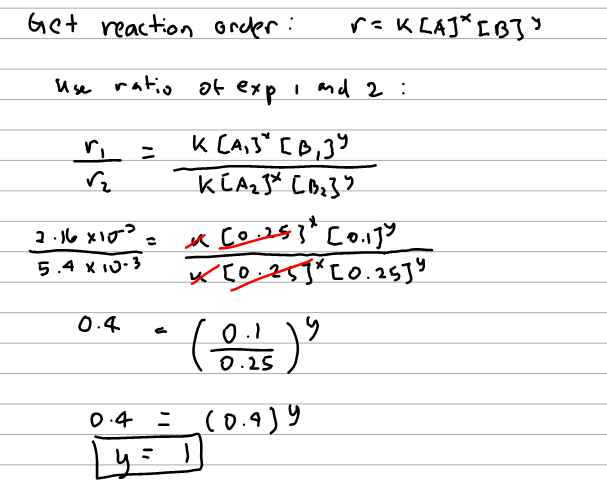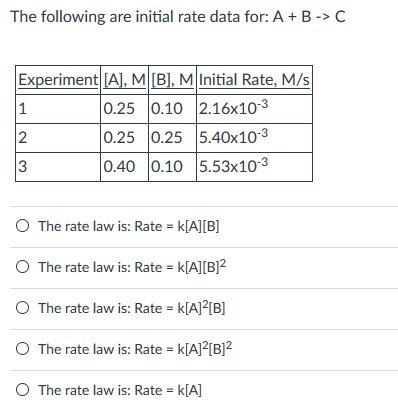# Problem: The following are initial rate data for: A + B → C (a) The rate law is: Rate = k[A][B] (b) The rate law is: Rate = k[A][B]2 (c) The rate law is: Rate = k[A]2[B] (d) The rate law is: Rate = k[A]2[B]2 (e) The rate law is: Rate = k[A]

###### FREE Expert Solution91% (327 ratings)###### Problem Details

The following are initial rate data for: A + B → C

(a) The rate law is: Rate = k[A][B]

(b) The rate law is: Rate = k[A][B]2

(c) The rate law is: Rate = k[A]2[B]

(d) The rate law is: Rate = k[A]2[B]2

(e) The rate law is: Rate = k[A]